# HLSL Development Cookbook

By Doron Feinstein
• \$30.99 eBook
• \$51.99 Print + eBook
What do you get with a Packt Subscription?

• Instant access to this title and 7,500+ eBooks & Videos
• Constantly updated with 100+ new titles each month
• Breadth and depth in over 1,000+ technologies

3D graphics are becoming increasingly more realistic and sophisticated as the power of modern hardware improves. The High Level Shader Language (HLSL) allows you to harness the power of shaders within DirectX 11, so that you can push the boundaries of 3D rendering like never before.

HLSL Development Cookbook will provide you with a series of essential recipes to help you make the most out of different rendering techniques used within games and simulations using the DirectX 11 API.

This book is specifically designed to help build your understanding via practical example. This essential Cookbook has coverage ranging from industry-standard lighting techniques to more specialist post-processing implementations such as bloom and tone mapping. Explained in a clear yet concise manner, each recipe is also accompanied by superb examples with full documentation so that you can harness the power of HLSL for your own individual requirements.

Publication date:
June 2013
Publisher
Packt
Pages
224
ISBN
9781849694209

## Chapter 1. Forward Lighting

In this chapter we will cover:

• Hemispheric ambient light

• Directional light

• Point light

• Spot light

• Capsule light

• Projected texture – point light

• Projected texture – spot light

• Multiple lights in a single pass

## Introduction

Forward lighting is a very common method to calculate the interaction between the various light sources and the other elements in the scene, such as meshes and particle systems. Forward lighting method has been around from the fixed pipeline days (when programmable shaders were just an insightful dream) till today, where it gets implemented using programmable shaders.

From a high-level view, this method works by drawing every mesh once for each light source in the scene. Each one of these draw calls adds the color contribution of the light to the final lit image shown on the screen. Performance wise, this is very expensive—for a scene with N lights and M meshes, we would need N times M draw calls. The performance can be improved in various ways. The following list contains the top four commonly used optimizations:

• Warming the depth buffer with all the fully opaque meshes (that way, we don't waste resources on rendering pixels that get overwritten by other pixels closer to the camera).

• Skip light sources and scene elements that are not visible to the camera used for rendering the scene.

• Do bounding tests to figure which light affects which mesh. Based on the results, skip light/mesh draw calls if they don't intersect.

• Combine multiple light sources that affect the same mesh together in a single draw call. This approach reduces the amount of draw calls as well as the overhead of preparing the mesh information for lighting.

Rendering the scene depths, as mentioned in the first method, is very easy to implement and only requires shaders that output depth values. The second and third methods are implemented on the CPU, so they won't be covered in this book. The fourth method is going to be explained at the end of this chapter. Since each one of these methods is independent from the others, it is recommended to use all of them together and gain the combined performance benefit.

Although this method lost its popularity in recent years to deferred lighting/shading solutions (which will be covered in the next chapter) and tiled lighting due to their performance improvement, it's still important to know how forward lighting works for the following reasons:

• Forward lighting is perfect for lighting scene elements that are not fully opaque. In fact, both deferred methods only handle opaque elements. This means that forward lighting is still needed for scenes containing translucent elements.

• Forward lighting can perform well when used for low-quality rendering tasks, such as low-resolution reflection maps.

• Forward lighting is the easiest way to light a scene, which makes it very useful for prototyping and in cases where real-time performance is not important.

All the following recipes are going to cover the HLSL side of the rendering. This means that you, the reader, will need to know how to do the following things:

• Prepare a system that will load and manage the scene

• Prepare a framework that supports Direct3D draw calls with shaders that will render the scene

All vertex buffers used with this technique must contain both positions and normals. In order to achieve smooth results, use smooth vertex normals (face normals should be avoided).

In addition, the pixel shader has to come up with a per-pixel color value for the rendered meshes. The color value may be a constant per mesh color or can be sampled from a texture.

## Hemispheric ambient light

Ambient light is the easiest light model to implement and yet it is very important to the overall look of your scene. For the most part, ambient light refers to any light in the scene that cannot be directly tied to a specific light source. This definition is flexible and its implementation will be shown soon.

In the past, a single constant color value was used for every mesh in the scene that provides a very flat result. As programmable shaders became more available, programmers switched from constant color to other solutions that take the mesh normal into account and avoid the flat look. Hemispheric lighting is a very common method to implement ambient lighting that takes normal values into account and does not require a lot of computations. The following screenshot shows the same mesh rendered with a constant ambient color (left-hand side) and with hemispheric lighting (right-hand side):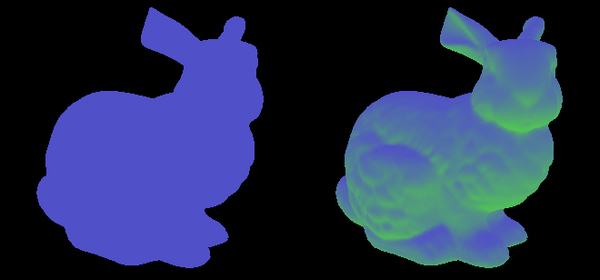As you can see, constant ambient light hides all the mesh detail, while hemispheric light provides a much more detailed result.

Hemispheric ambient light requires two colors that represent the light coming from above and below each mesh being rendered. We will be using a constant buffer to pass those colors to the pixel shader. Use the following values to fill a `D3D11_BUFFER_DESC` object:

Constant Buffer Descriptor Parameter

Value

`Usage`

`D3D11_USAGE_DYNAMIC`

`BindFlags`

`D3D11_BIND_CONSTANT_BUFFER`

`CPUAccessFlags`

`D3D11_CPU_ACCESS_WRITE`

`ByteWidth`

`8`

The reset of the descriptor fields should be set to `0`.

Creating the actual buffer, which is stored as a pointer to a `ID3D11Buffer` object, call the D3D device function `CreateBuffer` with the buffer-descriptor pointer as the first parameter, `NULL` as the second parameter, and a pointer to your `ID3D11Buffer` pointer as the last parameter.

### How to do it...

All lighting calculations are going to be performed in the pixel shader. This book assumes that you have the basic knowledge to set up and issue the draw call for each mesh in the scene. The minimum calculation a vertex shader has to perform for each mesh is to transform the position into projected space and the normal into world space.

### Note

If you are not familiar with the various spaces used in 3D graphics, you can find all the information you will need on Microsoft's MSDN at http://msdn.microsoft.com/en-us/library/windows/desktop/bb206269%28v=vs.85%29.aspx.

As a reference, the following vertex shader code can be used to handle those calculations:

```cbuffer cbMeshTrans : register( b0 )
{
float4x4  WorldViewProj  : packoffset( c0 );
float4x4  World    : packoffset( c4 );
}

struct VS_INPUT
{
float4 Pos  : POSITION;
float3 Norm  : NORMAL;
float2 UV  : TEXCOORD0;
};

struct VS_OUTPUT
{
float4 Pos  : SV_POSITION;
float2 UV  : TEXCOORD0;
float3 Norm  : TEXCOORD1;
};

VS_OUTPUT RenderSceneVS(VS_INPUT IN)
{
VS_OUTPUT Output;

// Transform position from object to projection space
Output.Pos = mul(IN.Pos, WorldViewProj);

// Copy the texture coordinate through
Output.UV = input.TextureUV;

// Transform normal from object to world space
Output.Norm = mul(IN.Norm, (float3x3)World);

return Output;
}```

### Tip

You can download the example code files for all Packt books you have purchased from your account at http://www.packtpub.com. If you purchased this book elsewhere, you can visit http://www.packtpub.com/support and register to have the files e-mailed directly to you.

Again, this code is for reference, so feel free to change it in any way that suits your needs.

In the pixel shader, we will use the following deceleration to access the values stored in the constant buffer:

```cbuffer HemiConstants : register( b0 )
{
float3 AmbientDown   : packoffset( c0 );
float3 AmbientRange  : packoffset( c1 );
}```

### Note

See the How it works... section for full details for choosing the values for these two constants.

Unless you choose to keep the two constant buffer values constant, you need to update the constant buffer with the values before rendering the scene. To update the constant buffer, use the context functions, `Map` and `Unmap`. Once the constant buffer is updated, bind it to the pixel shader using the context function `PSSetConstantBuffers`.

Our pixel shader will be using the following helper function to calculate the ambient value of a pixel with a given normal:

```float3 CalcAmbient(float3 normal, float3 color)
{
// Convert from [-1, 1] to [0, 1]
float up = normal.y * 0.5 + 0.5;
// Calculate the ambient value
float3 Ambient = AmbientDown + up * AmbientUp;

// Apply the ambient value to the color
return Ambient * color;
}```

This function assumes the normal `y` component is the up/down axis. If your coordinate system uses a different component as the vertical axis, change the code accordingly.

Similar to the vertex shader, the code for the pixel shader entry point depends on your specific mesh and requirements. As an example, the following code prepares the inputs and calls the helper function:

```// Normalize the input normal
float3 normal = normalize(IN.norm);

// Convert the color to linear space
color = float4(color.rgb * color.rgb, color.a);

// Call the helper function and return the value
return CalcAmbient(normal, color);```

### How it works...

In order to understand how ambient light works, it is important to understand the difference between how light works in real life and the way it works in computer graphics. In real life, light gets emitted from different sources such as light bulbs and the Sun. Some of the rays travel straight from the source to our eyes, but most of them will hit surfaces and get reflected from them in a different direction and with a slightly different wave length depending on the surface material and color. We call each time the light gets reflected from a surface to a bounce. Since each light bounce changes the wave length, after a number of bounces the wave length is no longer visible; so what our eyes see is usually the light that came straight from the source plus the light that bounced for a very small amount of times. The following screenshot demonstrates a situation where a light source emits three rays, one that goes directly to the eye, one that bounces once before it reaches the eye, and one that bounces twice before it reaches the eye: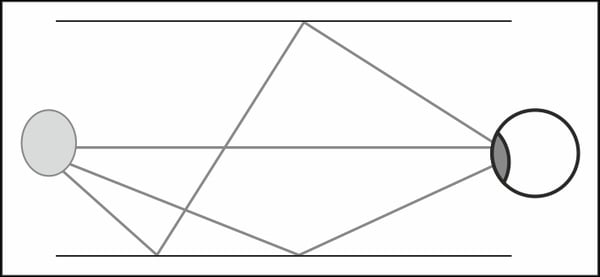In computer graphics, light calculation is limited to light that actually reaches the viewer, which is usually referred to as the camera. Calculating the camera's incoming light is normally simplified to the first bounce mostly due to performance restrictions. Ambient light is a term that usually describes any light rays reaching the camera that bounced from a surface more than once. In the old days, when GPUs where not programmable, ambient light was represented by a fixed color for the entire scene.

### Note

Graphics Processing Unit (GPU) is the electronic part in charge of graphics calculations. When a GPU is not programmable, we say that it uses a fixed pipeline. On the other hand, when a GPU is programmable, we say that it uses a programmable pipeline. DirectX 11-enabled cards are all programmable, so you are not likely to work with a fixed pipeline.

As the first screenshot in this recipe introduction showed, using a fixed color provides a flat and artificial look. As programmable GPUs became commonly available, developers finally had the flexibility to implement better the ambient light models that provide a more natural look. Although the hemispheric ambient light model is not a perfect representation of light that bounced more than once, it gained its popularity due to its simplicity and quality.

Hemispheric ambient light splits all light rays that affect the mesh getting rendered to those that arrived from above and below the mesh. Each one of these two directions is assigned a different color and intensity. To calculate the ambient light value of a given pixel, we use the normal vertical direction to linearly blend the two colors. As an example, in an outdoor scene with blue sky and grassy ground, the ambient light interpolates across the hemisphere, as shown in the following image: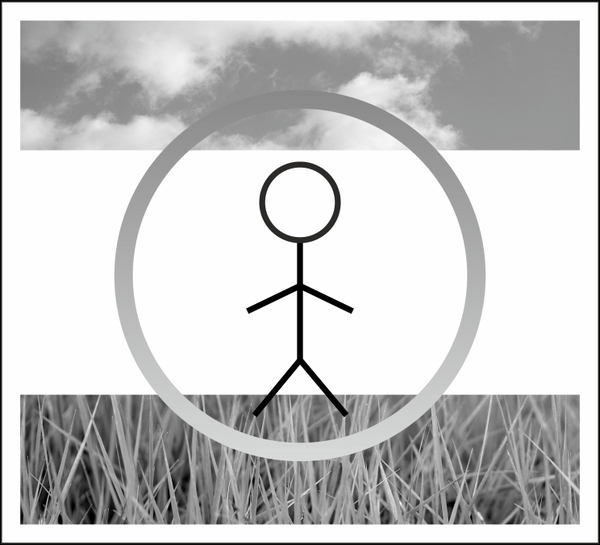Picking a pair of colors that properly represents the mesh surrounding for the upper and lower hemisphere is probably the most important step in this recipe. Though you can write code that picks the color pairs based on the scene and the camera position, in most games the values are handpicked by artists.

### Note

Note that even though the color pairs are constant for all the pixels affected by the draw call, they don't have to be constant overtime or for all the meshes in the scene. In fact, changing the color values based on the time of day or room properties is a very common practice.

One thing to keep in mind when picking the colors is the space they are in. When an artist manually picks a color value, he usually comes up with color values in what is known as gamma space. Light calculations on the other hand should always be performed in linear space. Any color in gamma space can be converted to linear space by raising it to the power of 2.2, but a faster and common approximation is to square the color (raising it to the power of 2). As you can see in the pixel shader entry point, we converted the pixel color to linear space before passing it to the ambient calculation.

### Tip

If you are not familiar with the gamma and linear color space, you should read about gamma correction to understand why it is so important to calculate lighting in linear space in the following link: http://www.slideshare.net/naughty_dog/lighting-shading-by-john-hable.

Once you picked the two values and converted them to linear space, you will need to store the colors in the constant buffer as the down color and the range to the upper color. In order to understand this step, we should look at the way the ambient color is calculated inside the helper function. Consider the following linear interpolation equation:

DownColor * (1-a) + UpColor * a = DownColor + a * (UpColor - DownColor)

The equation on the left-hand side blends the two colors based on the value, while the equation on the right-hand side does the exact same thing only with the down color and the range between the two colors. The GPU can handle the calculation on the right-hand side with a single instruction (this instruction is called madd), which makes it faster than the equation on the left-hand side. Since we use the equation on the left-hand side, you will need to store the upper, minus-lower color into the second constant buffer parameter.

## Directional light

Directional light is mainly used for simulating light coming from very large and far light sources, such as the sun and the moon. Because the light source is very large and far, we can assume that all light rays are parallel to each other, which makes the calculations relatively simple.

The following screenshot shows the same model we used to demonstrate ambient light under directional light: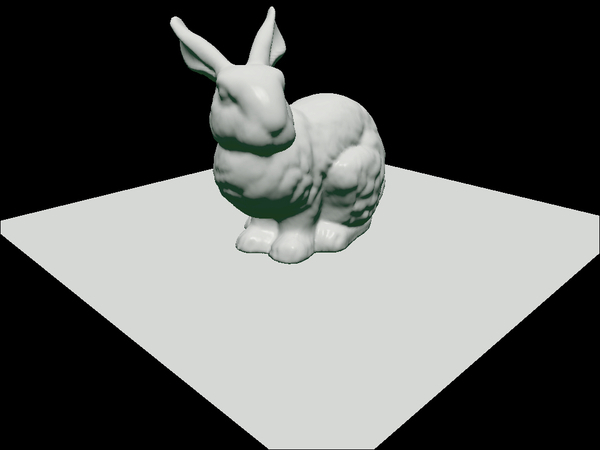When rendering an outdoor scene that uses directional light to represent the sun or the moon, it is very common to combine the directional light calculation with the ambient light calculation. However, you may still want to support ambient light with no directional light for indoor rendering. For this reason, we will allocate a separate constant buffer for the values used when calculating the directional light. Use the following values in the constant buffer descriptor:

Constant Buffer Descriptor Parameter

Value

`Usage`

`D3D11_USAGE_DYNAMIC`

`BindFlags`

`D3D11_BIND_CONSTANT_BUFFER`

`CPUAccessFlags`

`D3D11_CPU_ACCESS_WRITE`

`ByteWidth`

`8`

The reset of the descriptor fields should be set to `0`.

The three light values are needed for calculating the directional light: direction, intensity, and color. When rendering a scene with a fixed time of day, those values can be picked in advance by an artist. The only thing to keep in mind is that when this light source represents the Sun/Moon, the sky has to match the selected values (for example, low angle for the Sun means that the sky should show sunset/sunrise).

When time of day is dynamic, you will need multiple values for the different parts of the day/night cycle. An easy way to accomplish that is by picking values for a group of specific times in the day/night cycle (for instance, a value for every 3 hours in the cycle) and interpolate between those values based on the actual position in the cycle. Again, those values have to match the sky rendering.

To apply the light values on a given scene element, a few specific values are needed for the light calculation. Those scene element values will be referred to as the material. The material usually holds per-pixel values such as normal, diffuse color, and specular values. The material values can originate from texture samples or from global values.

### How to do it...

Similar to the ambient light, all directional, light-related calculations are handled in the pixel shader. We will be using the following constant buffer declaration in the shader for the new constant buffer:

```cbuffer DirLightConstants : register( b0 )
{
float3 DirToLight    : packoffset( c0 );float3 DirLightColor : packoffset( c1 );
}```

Although this may be counterintuitive, the direction used for directional light calculations is actually the inversed direction (direction to the light). To calculate that value, just negate the light direction. The inverted direction is stored in the first shader constant `DirToLight`.

The light intensity value is important when rendering to a high-dynamic range (HDR) target. HDR is a technique that calculates light values in a range wider than `0` to `1` (for more detail, check the HDR rendering recipe in Chapter 4, Postprocessing, about post processing).To improve performance, you should combine the light intensity value with the light color (make sure that you convert the color to linear space first). If you are not using an HDR target, make sure that the combined intensity and color value is lower than one. This combined light intensity and color is stored in the second shader constant `DirLightColor`.

The material is defined by the following structure:

```struct Material
{
float3 normal;
float4 diffuseColor;
float specExp;
float specIntensity;
};```

The material values should be prepared in the pixel shader before calling the function that calculates the final lit color of the pixel. The normals should be in world space and normalized. The diffuse value can be a constant color or a sample from a texture. When a material doesn't support specular highlights, just set `specExp` to `1` and `specIntensity` to `0`, otherwise use appropriate values based on the desired look (see explanation to specular light in the How it works... section of this recipe).

Here is the code for calculating the directional light value based on the input parameters:

```float3 CalcDirectional(float3 position, Material material)
{
// Phong diffuse
float NDotL = dot(DirToLight, material.normal);
float3 finalColor = DirLightColor.rgb * saturate(NDotL);

// Blinn specular
float3 ToEye = EyePosition.xyz - position;
ToEye = normalize(ToEye);
float3 HalfWay = normalize(ToEye + DirToLight);
float NDotH = saturate(dot(HalfWay, material.normal));
finalColor += DirLightColor.rgb * pow(NDotH, material.specExp) * material.specIntensity;

return finalColor * material.diffuseColor.rgb;
}```

This function takes the pixel's world position and material values, and it outputs the pixel's lit color value.

### How it works…

The Blinn-Phong light equation used in the previous code is very popular, as it is easy to compute and provides pleasing visual results. The equation is split into two components: a diffuse and a specular component. The following figure shows the different vectors used in the directional light calculation: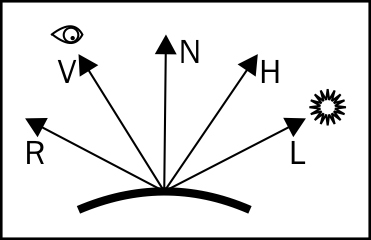Diffuse light is defined as a light reflected by the mesh surface equally in all directions. As you can see from the calculation, the diffuse light value for a given pixel is only affected by the normal N and by the direction to light L using the dot product value. If you recall from linear algebra, the dot product equals to:

Dot(N, L) = |N||L|cos(α)

Where α is the angle between N and L. Since all vectors are normalized, the size of N and the size of L is one, so the dot product in this case is equal to the cosine of the angle between the vectors. This means that the diffuse light gets brighter, as the normal N and the direction to the light L get closer to being parallel and dimmer as they get closer to being perpendicular.

Specular light, as opposed to diffuse light, gets reflected by the mesh in a specific direction. Light coming from the light source gets reflected in the direction R. Calculating the reflection vector R is a bit expensive, so Blinn's equation provides a very good and fast approximation using the half-way vector H (the vector at half the angle between the direction to the viewer V and the direction to the light L). If you imagine how the H light is going to move when V and L move, you will see that the angle between H and N gets smaller when the angle between R and V gets smaller. Using the dot product of N and H, we get a good estimate to how close the view direction is to the reflected vector R.

The power function is then used to calculate the intensity of the reflected light for the given angle between N and H. The higher the material's specular exponent is, the smaller the light spread is.

### There's more…

For performance reasons, it's very common to combine the ambient light calculation with the directional light in the same shader. In most scenes, there is only one directional light source, so by calculating both directional and ambient light in the same shader, you can save one draw call per mesh.

All you have to do is just add the value of the directional light to the ambient light value like this:

```// Calculate the ambient color
float4 finalColor;
finalColor.rgb = CalcAmbient(Normal, material.diffuseColor.rgb);

// Calculate the directional light
finalColor.rgb += CalcDirectional(worldPosition, material);```

## Point light

Point light is a light source that emits light equally in all directions. A good example for cases where a point light should be used is for an exposed light bulb, lit torch, and any other light source that emits light evenly in all directions.

The following screenshot shows the bunny with a point light in front of its chest: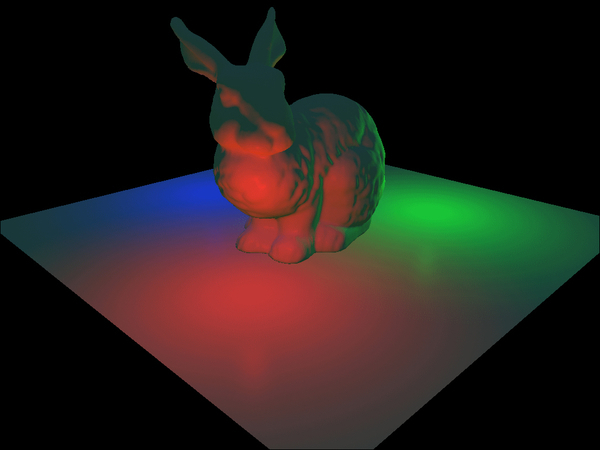Looking at the previous screenshot, you may be wondering why you can't see the actual light source. With the exception of an ambient light, all the light sources featured in this chapter only calculate the first light bounce. Because we don't calculate the effect of rays hitting the camera directly from the light source, the light source is invisible. It is a common practice to render a mesh that represents the light source with a shader that outputs the light's color of multiplayer by its intensity. This type of shader is commonly known as an emissive shader.

Point lights extend the directional light calculation by making the direction between each pixel and the light source based on the pixel and light position (unlike the fixed direction used in directional light).

Instead of the direction value used by directional light, point lights use a position and the range values. The position should be the center of the light source. The range should be the edge of the point light's influence (the furthest distance light can travel from the source and affect the scene).

### How to do it...

Similar to directional light, the point light is going to use the pixel position and the material structure. Remember that the normal has to be normalized and that the diffuse color has to be in linear space.

Instead of the direction vector used by directional light, point light requires a position in world space and a range in world space units. Inside the point light calculation, we need to divide the point lights range value. Since the GPU handles multiplication better than division, we store the Range value as 1/Range (make sure that the range value is bigger than zero), so we can multiply instead of divide.

### Note

1 / Range is called the reciprocal of Range.

We declare the position and reciprocal range inside the pixels header as follows:

```cbuffer DirLightConstants : register( b0 )
{
float3 PointLightPos  : packoffset( c0 );
float PointLightRangeRcp  : packoffset( c0.w );
}```

Here is the code for calculating the point light:

```float3 CalcPoint(float3 position, Material material)
{
float3 ToLight = PointLightPos.xyz - position;
float3 ToEye = EyePosition.xyz - position;
float DistToLight = length(ToLight);

// Phong diffuse
ToLight /= DistToLight; // Normalize
float NDotL = saturate(dot(ToLight, material.normal));
float3 finalColor = PointColor.rgb * NDotL;

// Blinn specular
ToEye = normalize(ToEye);
float3 HalfWay = normalize(ToEye + ToLight);
float NDotH = saturate(dot(HalfWay, material.normal));
finalColor += PointColor.rgb * pow(NDotH, material.specExp) * material.specIntensity;

// Attenuation
float DistToLightNorm = 1.0 - saturate(DistToLight * PointLightRangeRcp);
float Attn = DistToLightNorm * DistToLightNorm;
finalColor *= material.diffuseColor * Attn;

return finalColor;
}```

This function takes the pixel's world position and material values, and outputs the pixel's lit color value.

### How it works…

As with the directional light, the Blinn-Phong model is used for point light calculation. The main difference is that the light direction is no longer constant for all the pixels. Since the point light emits light in a sphere pattern, the light direction is calculated per pixel as the normalized vector from the pixel position to the light source position.

The attenuation calculation fades the light based on distance from the source. In the featured code, a squared attenuation is used. Depending on the desired look, you may find a different function more suitable.

### Tip

You can get a different attenuation value for each light source by using the HLSL pow function with a per-light source term.

## Spot light

Spot light is a light source that emits light from a given position in a cone shape that is rounded at its base. The following screenshot shows a spot light pointed at the bunny's head: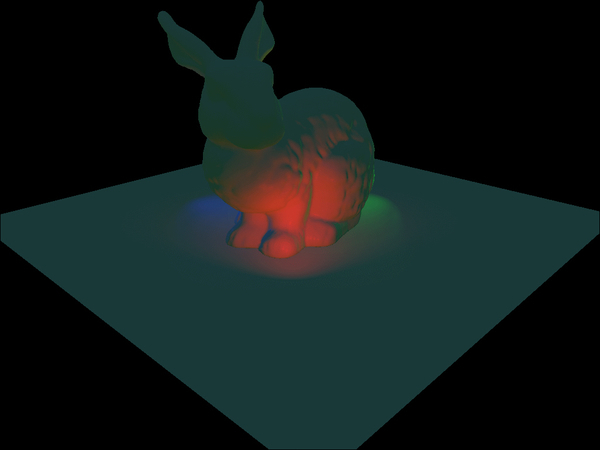The cone shape of the spot light is perfect for representing flash lights, vehicle's front lights, and other lights that are focused in a specific direction.

In addition to all the values needed for point light sources, a spot light has a direction and two angles to represent its cone. The two cone angles split the cone into an inner cone, where light intensity is even, and an outer cone, where light attenuates if it gets closer to the cone's border. The following screenshot shows the spot light direction as D, the inner to outer cone angle as α, and the outer cone angle as θ: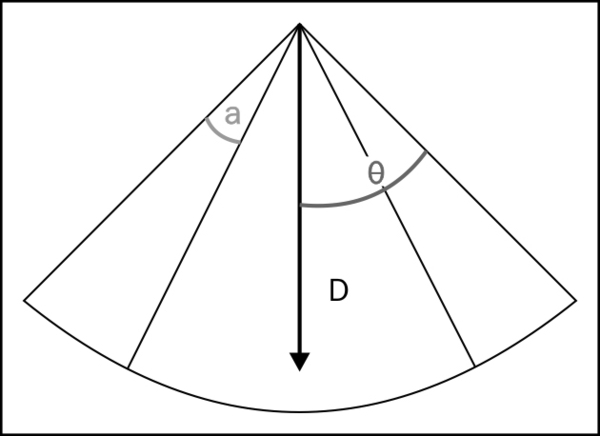Unlike the point light, where light intensity attenuates only over distance, spot lights intensity also attenuates across the angle α. When a light ray angle from the center is inside the range of α, the light gets dimmer; the dimmer the light, the closer the angle is to θ.

### How to do it...

For the spot light calculation, we will need all the values used for point light sources plus the additional three values mentioned in the previous section. The following deceleration contains the previous and new values:

```cbuffer SpotLightConstants : register( b0 )
{
float3 SpotLightPos      : packoffset( c0 );
float SpotLightRangeRcp  : packoffset( c0.w );
float3 SpotLightDir      : packoffset( c1 );
float SpotCosOuterCone   : packoffset( c1.w );
float SpotInnerConeRcp   : packoffset( c2 );
}```

Like the directional light's direction, the spot light's direction has to be normalized and inverted, so it would point to the light (just pass it to the shader, minus the light direction). The inverted direction is stored in the shader constant `SpotLightDir`.

Reciprocal range is stored in the shader constant `SpotLightRangeRcp`.

When picking the inner and outer cone angles, always make sure that the outer angle is bigger than the outer to inner angle. During the spot light calculation, we will be using the cosine of the inner and outer angles. Calculating the cosine values over and over for every lit pixel in the pixel shader is bad for performance. We avoid this overhead by calculating the cosine values on the CPU and passing them to the GPU. The two angle cosine values are stored in the shader constants `SpotCosOuterCone` and `SpotCosInnerCone`.

The code to calculate the spot light is very similar to the point light code:

```float3 CalcSpot(float3 position, Material material)
{
float3 ToLight = SpotLightPos - position;
float3 ToEye = EyePosition.xyz - position;
float DistToLight = length(ToLight);

// Phong diffuse
ToLight /= DistToLight; // Normalize
float NDotL = saturate(dot(ToLight, material.normal));
float3 finalColor = SpotColor.rgb * NDotL;

// Blinn specular
ToEye = normalize(ToEye);
float3 HalfWay = normalize(ToEye + ToLight);
float NDotH = saturate(dot(HalfWay, material.normal));
finalColor += SpotColor.rgb * pow(NDotH, material.specExp) * material.specIntensity;

// Cone attenuation
float conAtt = saturate((cosAng - SpotCosOuterCone) * SpotCosInnerConeRcp);
conAtt *= conAtt;

// Attenuation
float DistToLightNorm = 1.0 - saturate(DistToLight * SpotLightRangeRcp);
float Attn = DistToLightNorm * DistToLightNorm;
finalColor *= material.diffuseColor * Attn * conAtt;

return finalColor;
}```

As with the previous two light functions, this function takes the pixel's world position and material values, and outputs the pixel's lit color value.

### How it works…

As with the previous light sources, the spot light is using the Blinn-Phong model. The only difference in the code is the cone attenuation, which gets combined with the distance attenuation. To account for the cone shape, we first have to find the angle between the pixel to light vector and the light vector. For that calculation we use the dot product and get the cosine of that angle. We then subtract the cosine of the outer cone angle from that value and end up with one of the three optional results:

• If the result is higher than the cosine of the inner cone, we will get a value of `1` and the light affect will be fully on

• If the result is lower than the cosine of the inner cone but higher than zero, the pixel is inside the attenuation range and the light will get dimmer based on the size of the angle

• If the result is lower than zero, the pixel was outside the range of the outer cone and the light will not affect the pixel

## Capsule light

Capsule light, as the name implies, is a light source shaped as a capsule. Unlike spot and point light sources that have a point origin, the capsule light source has a line at its origin and it is emitting light evenly in all directions. The following screenshot shows a red capsule light source: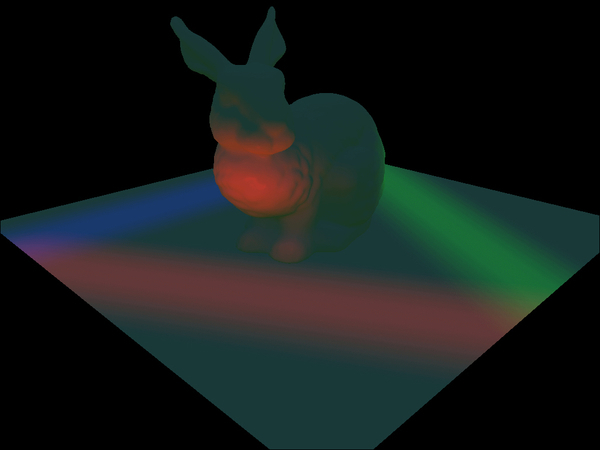Capsule lights can be used to represent fluorescent tubes or a lightsaber.

Capsules can be thought of as a sphere split into two halves, which are then extruded by the length of the capsule light's line length. The following figure shows the line start point A and end points B and R are the light's range: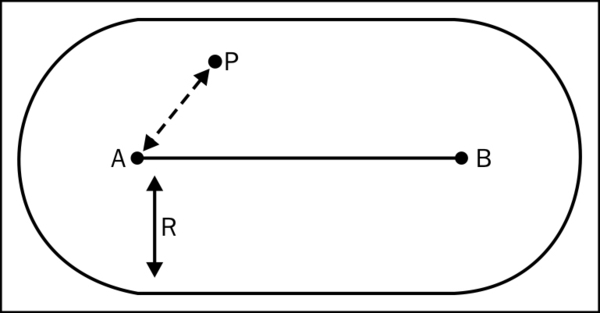### How to do it...

Capsule lights use the following constant buffer in their pixel shader:

```cbuffer CapsuleLightConstants : register( b0 )
{
float3 CapsuleLightPos     : packoffset( c0 );
float CapsuleLightRangeRcp : packoffset( c0.w );
float3 CapsuleLightDir     : packoffset( c1 );
float CapsuleLightLen      : packoffset( c1.w );
float3 CapsuleLightColor   : packoffset( c2 );
}```

Point A, referred to as the starting point is stored in the shader constant `CapsuleLightPos`.

In order to keep the math simple, instead of using the end point directly, we are going to use the normalized direction from A to B and the line's length (distance from point A to point B). We store the capsule's direction in the constant `CapsuleLightDir` and the length in `CapsuleLightLen`.

Similar to the point and spot lights, we store the range.

The code for calculating the capsule light looks like this:

```float3 CalcCapsule(float3 position, Material material)
{
float3 ToEye = EyePosition.xyz - position;

// Find the shortest distance between the pixel and capsules segment
float3 ToCapsuleStart = position - CapsuleLightPos;
float DistOnLine = dot(ToCapsuleStart, CapsuleDirLen.xyz) / CapsuleLightRange;
DistOnLine = saturate(DistOnLine) * CapsuleLightRange;
float3 PointOnLine = CapsuleLightPos + CapsuleLightDir * DistOnLine;
float3 ToLight = PointOnLine - position;
float DistToLight = length(ToLight);

// Phong diffuse
ToLight /= DistToLight; // Normalize
float NDotL = saturate(dot(ToLight, material.normal));
float3 finalColor = material.diffuseColor * NDotL;

// Blinn specular
ToEye = normalize(ToEye);
float3 HalfWay = normalize(ToEye + ToLight);
float NDotH = saturate(dot(HalfWay, material.normal));
finalColor += pow(NDotH, material.specExp) * material.specIntensity;

// Attenuation
float DistToLightNorm = 1.0 - saturate(DistToLight * CapsuleLightRangeRcp);
float Attn = DistToLightNorm * DistToLightNorm;
finalColor *= CapsuleLightColor.rgb * CapsuleIntensity * Attn;

return finalColor;
}```

This function takes the pixel's world position and material values, and it outputs the pixel's lit color value.

### How it works…

Look closely at the code and you will notice that it's basically the point light code except for the pixel to light position vector calculation. The idea is to find the closest point on the line to the pixel position. Once found, the vector to light is calculated by subtracting the closest position from the pixel position.

Finding the closest point on the line is done using the dot product. If you recall, the dot product result is the projected length of one vector over the other. By calculating the dot product of the vector AP with the capsule direction, we find the distance on the line from A to the closest point. We then have three possible results:

• The value is negative (outside the line from A's side); in this case the closest point is A

• The value is positive, but it's bigger than the line's length (outside the line from B's side); in this case the closest point is B

• The value is within the line's length and it doesn't need any modifications

HLSL is not very good with code branches, so instead of using `if` statements, the value found is normalized by dividing with the line's length and using the saturate instruction (clamp the value to zero and one). This affectively takes care of situations one and two. By multiplying the normalized value with the line's length, we end up with the correct distance from A. Now we can find the closest point by adding A and the distance of the capsule direction.

From that point on, the calculations are exactly the same as the point lights.

## Projected texture – point light

All light sources covered up to this point spread light in an even intensity distribution. However, sometimes a light source has a more sophisticated intensity distribution pattern. For example, a lamp shade can change the light intensity distribution and make it uneven. A different situation is when the intensity is even, but the color isn't due to something covering the light source. Using math to represent those and other situations may be too complex or have a negative effect on rendering performance. The most common solution in these cases is to use a texture that represents the intensity/color pattern emitted by these light sources.

The following screenshot shows a point light source projecting a texture with stars on the bunny: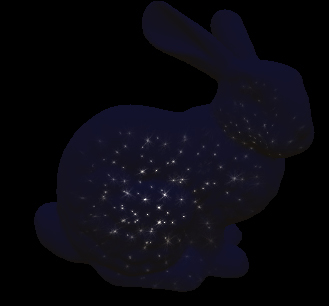To project a texture with a point light, you will need a texture that wraps around the point light's center. The best option is to use a cube map texture. The cube map texture is a group of six 2D textures that cover the faces of an imaginary box. Microsoft's Direct X SDK comes with a texture tool called DirectX Texture Tool, which helps you group six 2D textures and store them in an DXT format.

In order to sample the cube map texture, you will need a direction vector that points from the light source in the pixel directions. When the light is stationary, the texture can be prepared so the vector is the world-space direction from the light center to the pixel. If the light can rotate, the sampling direction has to take the rotation into an account. In those cases, you will need a matrix that transforms a direction in world space into the light's space.

Sampling the cube map texture will require a linear sampler state. Fill a `D3D11_SAMPLER_DESC` object with the following values:

Sampler State Descriptor Parameter

Value

`Fliter`

`D3D11_FILTER_MIN_MAG_MIP_LINEAR`

`AddressU`

`D3D11_TEXTURE_ADDRESS_WRAP`

`AddressV`

`D3D11_TEXTURE_ADDRESS_WRAP`

`AddressW`

`D3D11_TEXTURE_ADDRESS_WRAP`

`MaxAnisotropy`

`1`

`ComparisonFunc`

`D3D11_COMPARISON_ALWAYS`

`MaxLOD`

`D3D11_FLOAT32_MAX`

The reset of the descriptor fields should be set to `0`.

Create the actual sampler state from the descriptor using the device function `CreateSamplerState`.

### How to do it...

To keep the code generic, we are going to support light source rotation. For light sources that don't rotate, just use an identity matrix. For performance reasons, it is preferred to calculate the sampling direction in the vertex shader using the vertex world position.

In order to transform a position into light space, we are going to use the following shader constant matrix:

`float4x4 LightTransform;`

Usually when a light rotates, it is attached to some scene model that represents the light source. The model has a transformation from the model space to world space. All you need to do is inverse that transformation and use it as `LightTransform`.

Computing the sampling direction can be done in either the vertex or pixel shader using the following code (again, it is recommended to do this in the vertex shader):

```float3 GetDirToLight(float3 WorldPosition)
{
float3 ToLgiht = LightTransform.xyz + WorldPosition;
return mul(ToLgiht.xyz, (float3x3)LightTransform);
}```

This function takes the vertex/pixel position as argument and returns the sampling direction. If you choose to calculate the sampling direction in the vertex shader, make sure that you pass the result to the pixel shader.

In addition to the cube map texture, we will need a single intensity value. If you recall from the basic point light implementation, the intensity used to be combined with the color value. Now that the color value is sampled from a texture, it can no longer be combined with the intensity on the CPU. The intensity is stored in the following global variable:

`float PointIntensity;`

We will be accessing the cube map texture in the pixel shader using the following shader resource view deceleration:

`TextureCube ProjLightTex : register( t0 );`

As mentioned in the Getting ready section of this recipe, sampling the cube map will also require a linear sampler. Add the following sampler state deceleration in your pixel shader:

`SamplerState LinearSampler : register( s0 );`

Using the sampling direction, we can now find the per-pixel color value of the light using the following code:

```float3 GetLightColor(float3 SampleDirection)
{
return PointIntensity * ProjLightTex.Sample(LinearSampler, SampleDirection);
}```
```The returned color intensity should now be used to calculate color affect using the following code:
float3 CalcPoint(float3 LightColor, float3 position, Material material)
{
float3 ToLight = PointLightPos - position;
float3 ToEye = EyePosition.xyz - position;
float DistToLight = length(ToLight);

// Phong diffuse
ToLight /= DistToLight; // Normalize
float NDotL = saturate(dot(ToLight, material.normal));
float3 finalColor = LightColor * NDotL;

// Blinn specular
ToEye = normalize(ToEye);
float3 HalfWay = normalize(ToEye + ToLight);
float NDotH = saturate(dot(HalfWay, material.normal));
finalColor += LightColor * pow(NDotH, material.specExp) * material.specIntensity;
// Attenuation
float DistToLightNorm = 1.0 - saturate(DistToLight * PointLightRangeRcp);
float Attn = DistToLightNorm * DistToLightNorm;
finalColor *= material.diffuseColor * Attn;

return finalColor;
}```

If you compare this code with the code used for spot lights that don't use projected textures, you will notice that the only difference is the light color getting passed as an argument instead of the constant color used in the basic implementation.

### How it works…

We consider the cube map texture to be in light space. This means that the values in the texture stay constant regardless of the light rotation and movement. By transforming the direction to the light into light space, we can sample the texture with it and get the color value. Aside from the sampled color value replacing the constant color from the basic point light implementation, the code stays exactly the same.

## Projected texture – spot light

Similar to point lights with projected textures, spot lights can use a 2D texture instead of a constant color value. The following screenshot shows a spot light projecting a rainbow pattern on the bunny: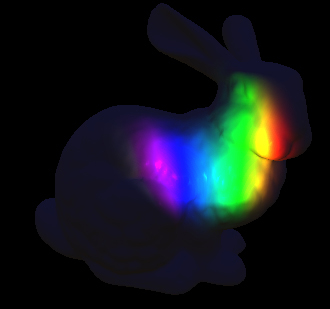Due to the cone shape of the spot light, there is no point in using a cube map texture. Most spot light sources use a cone opening angle of 90 degrees or less, which is equivalent to a single cube map face. This makes using the cube map a waste of memory in this case. Instead, we will be using a 2D texture.

Projecting a 2D texture is a little more complicated compared to the point light. In addition to the transformation from world space to light space, we will need a projection matrix. For performance reasons, those two matrices should be combined to a single matrix by multiplying them in the following order:

FinalMatrix = ToLightSpaceMatrix * LightProjectionMatrix

Generating this final matrix is similar to how the matrices used for rendering the scene get generated. If you have a system that handles the conversion of camera information into matrices, you may benefit from defining a camera for the spot light, so you can easily get the appropriate matrices.

### How to do it...

Spot light projection matrix can be calculated in the same way the projection matrix is calculated for the scene's camera. If you are unfamiliar with how this matrix is calculated, just use the following formulas: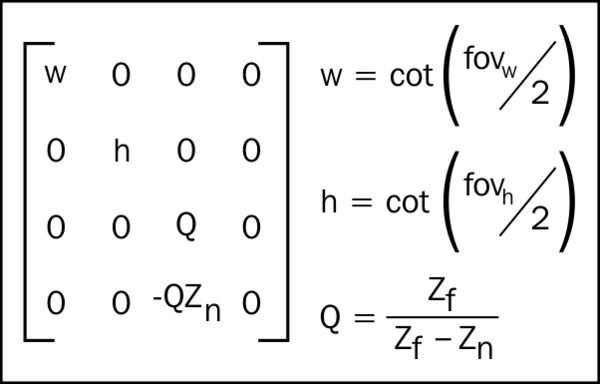In our case, both w and h are equal to the cotangent of the outer cone angle. Zfar is just the range of the light source. Znear was not used in the previous implementation and it should be set to a relatively small value (when we go over shadow mapping, this value's meaning will become clear). For now just use the lights range times 0.0001 as Znear's value.

The combined matrix should be stored to the vertex shader constant:

`float4x4 LightViewProjection;`

Getting the texture coordinate from the combined matrix is handled by the following code:

```float2 GetProjPos(float4 WorldPosition)
{
float3 ProjTexXYW = mul(WorldPosition, LightViewProjection).xyw;
ProjTexXYW.xy /= ProjTexXYW.z; // Perspective correction
Float2 UV = (ProjTexXYW.xy + 1.0.xx) * float2(0.5, -0.5); // Convert to normalized space
return UV;
}```

This function takes the world position as four components (`w` should be set to `1`) as parameter and returns the projected texture UV sampling coordinates. This function should be called in the vertex shader.

The texture coordinates should be than passed to the pixel shader, so they can be used for sampling the texture. In order to sample the texture, the following shader resource view should be defined in the pixel shader:

`Texture2D ProjLightTex : register( t0 );`

Sampling the texture is done in the pixel shader with the following code:

```float3 GetLightColor(float2 UV)
{
return SpotIntensity * ProjLightTex.Sample(LinearSampler, UV);
}```

This function takes the UV coordinates as parameter and returns the light's color intensity for the pixel. Similar to point lights with projected textures, the color sampled from the texture is then multiplied by the intensity to get the color intensity value used in the lighting code.

The only thing left to do is to use the light color intensity and light the mesh. This is handled by the following code:

```float3 CalcSpot(float3 LightColor, float3 position, Material material)
{
float3 ToLight = SpotLightPos - position;
float3 ToEye = EyePosition.xyz - position;
float DistToLight = length(ToLight);

// Phong diffuse
ToLight /= DistToLight; // Normalize
float NDotL = saturate(dot(ToLight, material.normal));
float3 finalColor = LightColor * NDotL;

// Blinn specular
ToEye = normalize(ToEye);
float3 HalfWay = normalize(ToEye + ToLight);
float NDotH = saturate(dot(HalfWay, material.normal));
finalColor += LightColor * pow(NDotH, material.specExp) * material.specIntensity;

// Cone attenuation
float cosAng = dot(SpotLightDir, ToLight);
float conAtt = saturate((cosAng - SpotCosOuterCone) / SpotCosInnerCone);
conAtt *= conAtt;

// Attenuation
float DistToLightNorm = 1.0 - saturate(DistToLight / SpotLightRange);
float Attn = DistToLightNorm * DistToLightNorm;
finalColor *= material.diffuseColor * Attn * conAtt;

return finalColor;
}```

Similar to the point light implementation with projected texture support, you will notice that the only difference compared to the basic spot light code is the light color getting passed as an argument.

### How it works…

Converting the world space position to texture coordinates is very similar to the way world positions get converted to the screen's clip space by the GPU. After multiplying the world position with the combined matrix, the position gets converted to projected space that can be then converted to clip space (`X` and `Y` values that are inside the lights influence will have the value `-1` to `1`). We then normalize the clip space values (`X` and `Y` range becomes `0` to `1`), which are the UV range we need texture sampling for.

All values passed to the vertex shader are linearly interpolated, so the values passed from the vertex shader to the pixel shader will be interpolated correctly for each pixel based on the UV values calculated for the three vertices, which make the triangle the pixel was rasterized from.

## Multiple light types in a single pass

In this chapter's introduction, we saw that the performance can be optimized by combining multiple lights into a single draw call. The GPU registers are big enough to contain four float values. We can take advantage of that size and calculate four lights at a time.

Unfortunately, one drawback of this approach is the lack of support for projected textures. One way around this issue is to render light sources that use projected textures separately from the rest of the light sources. This limit may not be all that bad depending on the rendered scene light setup.

Unlike the previous examples, this time you will have to send light data to the GPU in groups of four. Since it's not likely that all four light sources are going to be of the same type, the code handles all three light types in a generic way. In cases where the drawn mesh is affected by less than four lights, you can always disable lights by turning their color to fully black.

### How to do it...

In order to take full advantage of the GPU's vectorized math operations, all the light source values are going to be packed in groups of four. Here is a simple illustration that explains how four-three component variables can be packed into three-four component variables:All variable packing should be done on the CPU. Keeping in mind that the constant registers of the GPU are the size of four floats, this packing is more efficient compared to the single light version, where most of the values use only three floats and waste the forth one.

Light positions X, Y, and Z components of each of the four light sources are packed into the following shader constants:

```float4 LightPosX;
float4 LightPosY;
float4 LightPosZ;```

Light directions are separated to X, Y, and Z components as well. This group of constants is used for both, spot and capsule light source directions. For point lights make sure to set the respected value in each constant to `0`:

```float4 LightDirX;
float4 LightDirY;
float4 LightDirZ;```

Light color is separated to R, G, and B components. For disabled light sources just set the respected values to `0`:

```float4 LightColorR;
float4 LightColorG;
float4 LightColorB;```

As before, you should combine the color and intensity of each light before passing the values to the GPU.

All four light ranges are stored in a single four-component constant:

`float4 LightRange;`

All four lights' capsule lengths are stored in a single four-component constant. For noncapsule lights just store the respected value to `0`:

`float4 CapsuleLen;`

Spot light's cosine outer cone angle is again stored in a four-component constant. For nonspot light sources set the respected value to `-2`:

`float4 SpotCosOuterCone;`

Unlike the single spot light, for the inner cone angle we are going to store one over the spot light's cosine inner cone angle. For nonspot light sources set the respected value to `1`:

`float4 SpotCosInnerConeRcp;`

We are going to use two new helper functions that will help us calculate the dot product of four component vectors. The first one calculates the dot product between two groups of three-four component variable. The return value is a four-component variable with the four-dot product values. The code is as follows:

```float4 dot4x4(float4 aX, float4 aY, float4 aZ, float4 bX, float4 bY, float4 bZ)
{
return aX * bX + aY * bY + aZ * bZ;
}```

The second helper function calculates the dot product of three-four component variables with a single three-component variable:

```float4 dot4x1(float4 aX, float4 aY, float4 aZ, float3 b)
{
return aX * b.xxxx + aY * b.yyyy + aZ * b.zzzz;
}```

Finally, the code to calculate the lighting for the four light sources is as follows:

```   float3 ToEye = EyePosition.xyz - position;

// Find the shortest distance between the pixel and capsules segment
float4 ToCapsuleStartX = position.xxxx - LightPosX;
float4 ToCapsuleStartY = position.yyyy - LightPosY;
float4 ToCapsuleStartZ = position.zzzz - LightPosZ;
float4 DistOnLine = dot4x4(ToCapsuleStartX, ToCapsuleStartY, ToCapsuleStartZ, LightDirX, LightDirY, LightDirZ);
float4 CapsuleLenSafe = max(CapsuleLen, 1.e-6);
DistOnLine = CapsuleLen * saturate(DistOnLine / CapsuleLenSafe);
float4 PointOnLineX = LightPosX + LightDirX * DistOnLine;
float4 PointOnLineY = LightPosY + LightDirY * DistOnLine;
float4 PointOnLineZ = LightPosZ + LightDirZ * DistOnLine;
float4 ToLightX = PointOnLineX - position.xxxx;
float4 ToLightY = PointOnLineY - position.yyyy;
float4 ToLightZ = PointOnLineZ - position.zzzz;
float4 DistToLightSqr = dot4x4(ToLightX, ToLightY, ToLightZ, ToLightX, ToLightY, ToLightZ);
float4 DistToLight = sqrt(DistToLightSqr);

// Phong diffuse
ToLightX /= DistToLight; // Normalize
ToLightY /= DistToLight; // Normalize
ToLightZ /= DistToLight; // Normalize
float4 NDotL = saturate(dot4x1(ToLightX, ToLightY, ToLightZ, material.normal));
//float3 finalColor = float3(dot(LightColorR, NDotL), dot(LightColorG, NDotL), dot(LightColorB, NDotL));

// Blinn specular
ToEye = normalize(ToEye);
float4 HalfWayX = ToEye.xxxx + ToLightX;
float4 HalfWayY = ToEye.yyyy + ToLightY;
float4 HalfWayZ = ToEye.zzzz + ToLightZ;
float4 HalfWaySize = sqrt(dot4x4(HalfWayX, HalfWayY, HalfWayZ, HalfWayX, HalfWayY, HalfWayZ));
float4 NDotH = saturate(dot4x1(HalfWayX / HalfWaySize, HalfWayY / HalfWaySize, HalfWayZ / HalfWaySize, material.normal));
float4 SpecValue = pow(NDotH, material.specExp.xxxx) * material.specIntensity;
//finalColor += float3(dot(LightColorR, SpecValue), dot(LightColorG, SpecValue), dot(LightColorB, SpecValue));

// Cone attenuation
float4 cosAng = dot4x4(LightDirX, LightDirY, LightDirZ, ToLightX, ToLightY, ToLightZ);
float4 conAtt = saturate((cosAng - SpotCosOuterCone) * SpotCosInnerConeRcp);
conAtt *= conAtt;

// Attenuation
float4 DistToLightNorm = 1.0 - saturate(DistToLight * LightRangeRcp);
float4 Attn = DistToLightNorm * DistToLightNorm;
Attn *= conAtt; // Include the cone attenuation

// Calculate the final color value
float4 pixelIntensity = (NDotL + SpecValue) * Attn;
float3 finalColor = float3(dot(LightColorR, pixelIntensity), dot(LightColorG, pixelIntensity), dot(LightColorB, pixelIntensity));
finalColor *= material.diffuseColor;

return finalColor;```

### How it works…

Though this code is longer than the one used in the previous recipes, it basically works in the exact same way as in the single light source case. In order to support the three different light sources in a single code path, both the capsule light's closest point to line and the spot lights cone attenuation are used together.

If you compare the single light version of the code with the multiple lights version, you will notice that all the operations are done in the exact same order. The only change is that each operation that uses the packed constants has to be executed three times and the result has to be combined into a single four-component vector.

### There's more…

Don't think that you are limited to four lights at a time just because of the GPU's constant size. You can rewrite `CalcFourLights` to take the light constant parameters as inputs, so you could call this function more than once in a shader.

Some scenes don't use all three light types. You can remove either the spot or capsule light support if those are not needed (point lights are at the base of the code, so those have to be supported). This will reduce the shader size and improve performance.

Another possible optimization is to combine the ambient, directional, and multiple lights code into a single shader. This will reduce the total amount of draw calls needed and will improve performance.

### About the Author

• ##### Doron Feinstein

Doron Feinstein has been working as a graphics programmer over the past decade in various industries. Since he graduated his first degree in software engineering, he began his career working on various 3D simulation applications for military and medical use. Looking to fulfill his childhood dream, he moved to Scotland for his first job in the game industry at Realtime Worlds. Currently working at Rockstar Games as a Senior Graphics Programmer, he gets to work on the company’s latest titles for Xbox 360, PS3, and PC.

Browse publications by this author